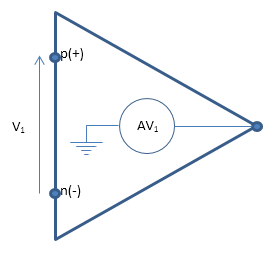# Op-Amp

Ideal operational amplifier

## Library

Electrical Elements

•## Description

The Op-Amp block models an ideal operational amplifier (op-amp). The figure shows the implementation schematic.The block implementation is based on the following assumptions:

• The ideal op-amp gain A is assumed to be infinite

• Then, for finite output, must have V1 = 0

• Ideal op-amp also implies current from p to n is zero (i1 = 0)

These assumptions result in the following equations for the block:

```equations v1 == p.v - n.v; v1 == 0; i1 == 0; end```

For more information, click the Source code link in the block dialog box.

You can initialize the Current into output node variable prior to simulation. For more information, see Block-Level Variable Initialization.

## Ports

The block has three electrical conserving ports.

## Version History

Introduced in R2007a